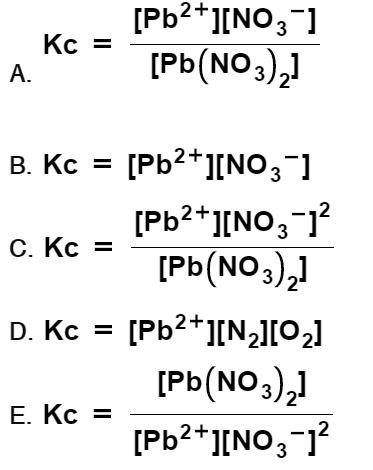Chemistry Practice Problems Equilibrium Expressions Practice Problems Solution: Which is the correct Kc expression for the followi...

# Solution: Which is the correct Kc expression for the following equilibrium reaction?Pb(NO3)2(aq) ⇌ Pb2+(aq) + 2 NO3–(aq)

###### Problem

Which is the correct Kc expression for the following equilibrium reaction?

Pb(NO3)2(aq) ⇌ Pb2+(aq) + 2 NO3(aq)###### Solution

Setup the Kc expression for the reaction and determine which is the correct one for the reaction:

Pb(NO3)2(aq)  Pb2+(aq) + 2 NO3(aq)

Kc is the equilibrium expression of the concentrations in the reaction. The general form of an equilibrium expression is:View Complete Written Solution

Equilibrium Expressions

Equilibrium Expressions

#### Q. At 700 K, the reaction below has an Kp value of 54. An equilibrium mixture at thistemperature was found to contain 1.49 atm of I2 and 4.08 atm of HI. ...

Solved • Thu Dec 12 2019 11:43:56 GMT-0500 (EST)

Equilibrium Expressions

#### Q. At 700 K, the reaction below has a Kp value of 54. An equilibrium mixture at thistemperature was found to contain 1.84 atm of I2 and 4.24 atm of HI. C...

Solved • Wed Dec 11 2019 14:53:32 GMT-0500 (EST)

Equilibrium Expressions

#### Q. Which is the correct Kc expression for the following equilibrium reaction?BaO(s) + CO2(g) ⇌ BaCO3(s)

Solved • Wed Nov 13 2019 13:20:48 GMT-0500 (EST)

Equilibrium Expressions

#### Q. At 500.0 K, 1.0 mole of gaseous ONCl is placed in a 1.0-liter container. At equilibrium, it is 9.0% dissociated according to the equation:2 ONCl(g) ⇌ ...

Solved • Tue Nov 12 2019 15:06:26 GMT-0500 (EST)